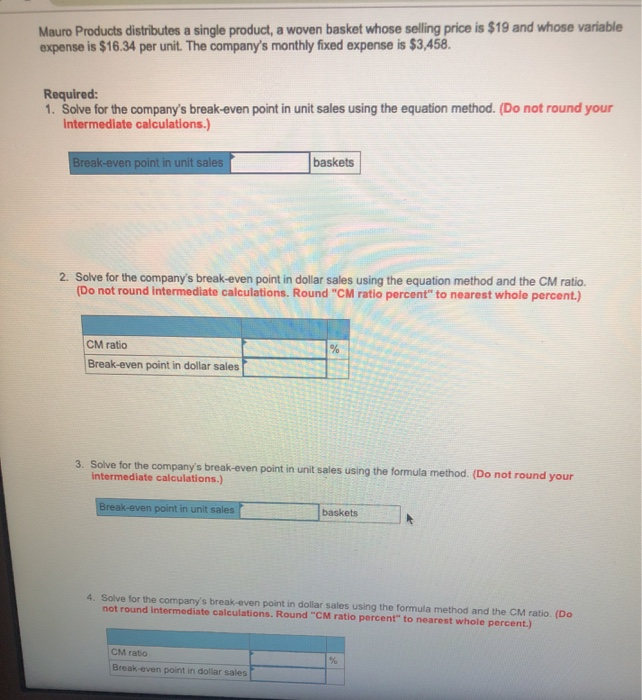# Mauro Products distributes a single product, a woven basket whose selling price is \$19 and whose variable expense i...Mauro Products distributes a single product, a woven basket whose selling price is \$19 and whose variable expense is \$16.34 per unit. The company's monthly fixed expense is \$3,458. Required: 1. Solve for the company's break-even point in unit sales using the equation method. (Do not round your intermediate calculations.) baskets ak-even point in unit sales 2. Solve for the company's break-even point in dollar sales using the equation method and the CM ratio. (Do not round intermediate calculations. Round "CM ratio percent" to nearest whole percent.) CM ratio Break-even point in dollar sales 3. Solve for the company's break-even point in unit sales using the formula method. (Do not round your intermediate calculations.) Break-even point in unit sales baskets 4. Solve for the company's break-even point in dollar sales using the formula method and the CM ratio. (Do not round Intermediate calculations. Round "CM ratio percent" to nearest whole percent.) CM ratio Break even point in dollar sales

Let breakeven point in units be x

Profit = Sales - Variable expenses - Fixed expenses
0 = \$19 * x - \$16.34 * x - \$3,458
\$2.66 * x = \$3,458
x = 1,300 units

Breakeven point in unit sales = 1,300 units

Contribution margin ratio = (Selling price per unit - Variable cost per unit) / Selling price per unit
Contribution margin ratio = (\$19.00 - \$16.34) / \$19.00
Contribution margin ratio = 14%

Let breakeven point in dollar sales be \$x

Profit = Sales * Contribution margin ratio - Fixed expenses
0 = \$x * 0.14 - \$3,458
\$3,458 = \$x * 0.14
\$x = \$24,700

Breakeven point in dollar sales = \$24,700

Contribution margin per unit = Selling price per unit - Variable cost per unit
Contribution margin per unit = \$19.00 - \$16.34
Contribution margin per unit = \$2.66

Breakeven point in unit sales = Fixed expenses / Contribution margin per unit
Breakeven point in unit sales = \$3,458 / \$2.66
Breakeven point in unit sales = 1,300

Breakeven point in unit sales = 1,300 units

Contribution margin ratio = (Selling price per unit - Variable cost per unit) / Selling price per unit
Contribution margin ratio = (\$19.00 - \$16.34) / \$19.00
Contribution margin ratio = 14%

Breakeven point in unit sales = Fixed expenses / Contribution margin ratio
Breakeven point in unit sales = \$3,458 / 0.14
Breakeven point in unit sales = \$24,700

Breakeven point in dollar sales = \$24,700

##### Add Answer of: Mauro Products distributes a single product, a woven basket whose selling price is \$19 and whose variable expense i...
More Homework Help Questions Additional questions in this topic.